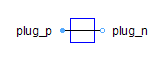Constant Magnetic Potential Difference - MapleSim Help

Home : Support : Online Help : MapleSim : MapleSim Component Library : Magnetic : Sources : Constant Magnetic Potential Difference

Constant Magnetic Potential Difference

Constant magnetomotive forceDescription The Constant Magnetic Potential Difference component models a constant magnetomotive force.Equations $\mathrm{\Phi }={\mathrm{\Phi }}_{p}=-{\mathrm{\Phi }}_{n}$ ${V}_{m}={V}_{{m}_{p}}-{V}_{{m}_{n}}$Variables

 Name Units Description Modelica ID $\mathrm{\Phi }$ $\mathrm{Wb}$ Magnetic flux from port_p to port_n PhiConnections

 Name Description Modelica ID ${\mathrm{port}}_{p}$ Positive magnetic port port_p ${\mathrm{port}}_{n}$ Negative magnetic port port_nParameters

 Name Default Units Description Modelica ID ${V}_{m}$ $A$ Magnetic potential difference V_mModelica Standard Library The component described in this topic is from the Modelica Standard Library. To view the original documentation, which includes author and copyright information, click here.